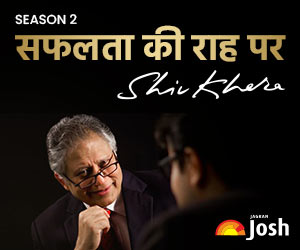# IIT JEE 2012 ANALYSIS & Question Paper of PHYSICS

Candidates can download the IIT JEE 2012 Question Paper and Complete Analysis of the paper.IIT JEE 2012 ANALYSIS & Question Paper of PHYSICS:
IIT JEE is one of the toughest examinations in the world. In the year 2012 this exam was conducted in two parts, first was PAPER-1 and second was PAPER-2. There were 20 questions of each subject in a paper and each paper consists of 60 questions in total. Paper-1 was of 210 marks and Paper-2 was of 198 marks. This whole booklet is very useful from exam point of view. This booklet consists of three different parts.

• First part consists of the analysis of the PHYSICS part in Paper-1 and Paper-2. Analysis is very helpful in many aspects; it will help you in understanding:
1. Importance of chapters.
3. Trend of the papers.
• Second part contains all the questions asked in PHYSICS in Paper-1 and Paper-2 with the answers. This Part will help you in understanding the difficulty level of the exam.
• Third and the last part contain the marking scheme and type of questions asked in the question paper.

QUESTIONS:Qu. A person blows into open-end of a long pipe. As a result, a high pressure pulse of air travels down the pipe. When this pulse reaches the other end of the pipe,
(A) A high-pressure pulse starts travelling up the pipe, if the other end of the pipe is open.
(B) A low-pressure pulse starts travelling up the pipe, if the other end of the pipe is open.
(C) A low-pressure pulse starts travelling up the pipe, if the other end of the pipe is closed.
(D) A high-pressure pulse starts travelling up the pipe, if the other end of the pipe is closed.

Ans. (B, D)

Qu. Two solid cylinders P and Q of same mass and same radius start rolling down a fixed inclined plane from the same height at the same time. Cylinder P has most of its mass concentrated near its surface, while Q has most of its mass concentrated near the axis. Which statement(s) is (are) correct?
(A) Both cylinders P and Q reach the ground at the same time.
(B) Cylinder P has larger linear acceleration than cylinder Q.
(C) Both cylinders reach the ground with same translational kinetic energy.
(D) Cylinder Q reaches the ground with larger angular speed.

Ans. (D)

Qu. A current carrying infinitely long wire is kept along the diameter of a circular wire loop, without touching it, the correct statement(s) is(are):
(A) The emf induced in the loop is zero if the current is constant.
(B) The emf induced in the loop is finite if the current is constant.
(C) The emf induced in the loop is zero if the current decreases at a steady rate.
(D) The emf induced in the loop is infinite if the current decreases at a steady rate.

Ans. (A, C)